# How to Calculate and Solve for Magnetic Flux | Magnetic Field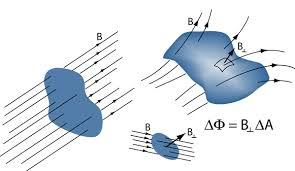The image above represents magnetic flux.

To compute for magnetic flux, two essential parameters are needed and these parameters are Area (A) and Total Magnetic Field (B).

The formula for calculating magnetic flux:

φ = B.A

Where:

φ = Magnetic Flux
A = Area
B = Total Magnetic Field

Let’s solve an example;
Find the magnetic flux with a given area of 32 and a total magnetic field of 18.

This implies that;

A = Area = 32
B = Total Magnetic Field = 18

φ = B.A
φ = (18)(32)
φ = 576

Therefore, the magnetic flux is 576 Wb.

Calculating the Area when the Magnetic Flux and the Total Magnetic Field is Given.

A = φ / B

Where;

A = Area
φ = Magnetic Flux
B = Total Magnetic Field

Let’s solve an example;
Find the area when the magnetic flux is 40 and the total magnetic field is 20.

This implies that;

φ = Magnetic Flux = 40
B = Total Magnetic Field = 20

A = φ / B
A = 40 / 20
A = 2

Therefore, the area is 2.

Calculating the Total Magnetic Field when the Magnetic Flux and the Area is Given.

B = φ / A

Where;

B = Total Magnetic Field
φ = Magnetic Flux
A = Area

Let’s solve an example;
Find the total magnetic field when the magnetic flux is 25 and the area is 5.

This implies that;

φ = Magnetic Flux = 25
A = Area = 5

B = φ / A
B = 25 / 5
B = 5

Therefore, the total magnetic field is 5.

Nickzom Calculator – The Calculator Encyclopedia is capable of calculating the magnetic flux.

To get the answer and workings of the magnetic flux using the Nickzom Calculator – The Calculator Encyclopedia. First, you need to obtain the app.

You can get this app via any of these means:

To get access to the professional version via web, you need to register and subscribe for NGN 2,000 per annum to have utter access to all functionalities.
You can also try the demo version via https://www.nickzom.org/calculator

Apple (Paid) – https://itunes.apple.com/us/app/nickzom-calculator/id1331162702?mt=8
Once, you have obtained the calculator encyclopedia app, proceed to the Calculator Map, then click on Geology under Add-on.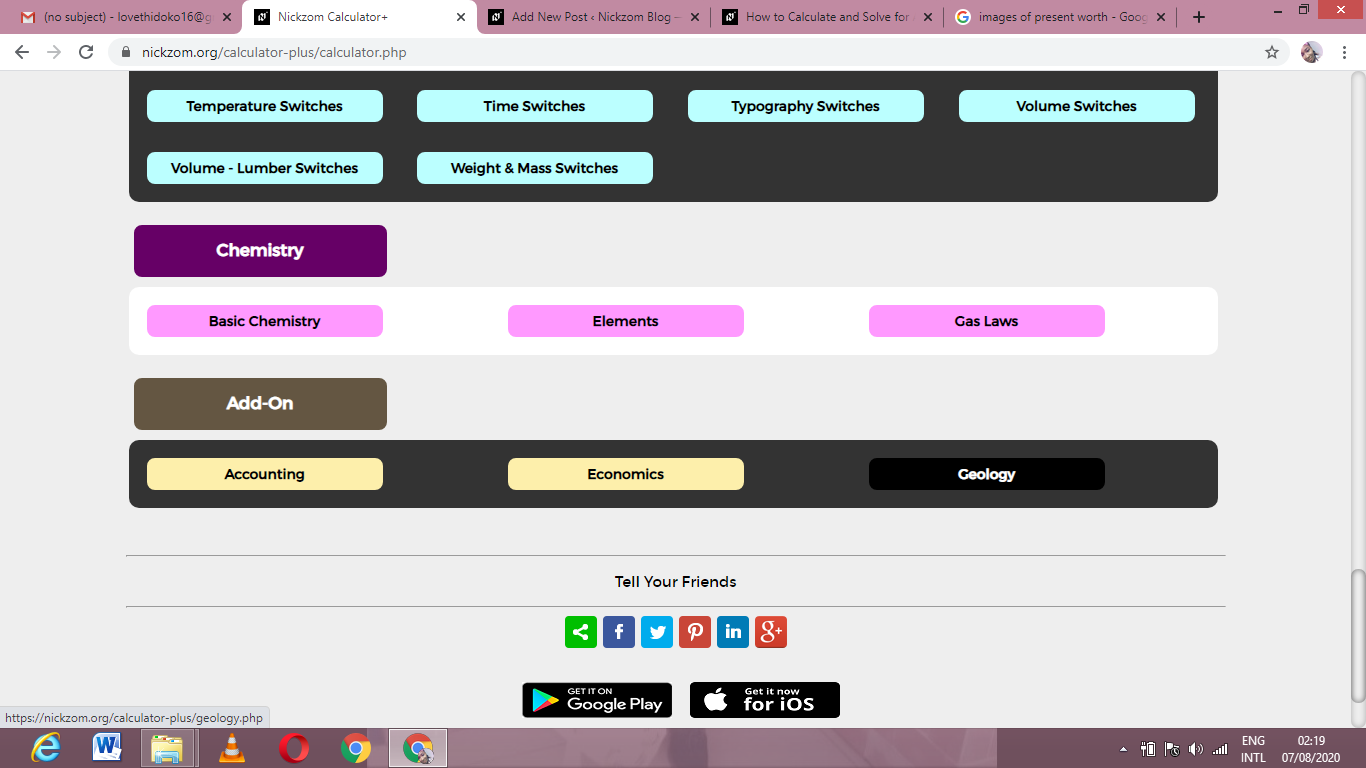Now, Click on Magnetic Field under GeologyNow, Click on Magnetic Flux under Magnetic FieldThe screenshot below displays the page or activity to enter your values, to get the answer for the magnetic flux according to the respective parameters which are the Area (A) and Total Magnetic Field (B).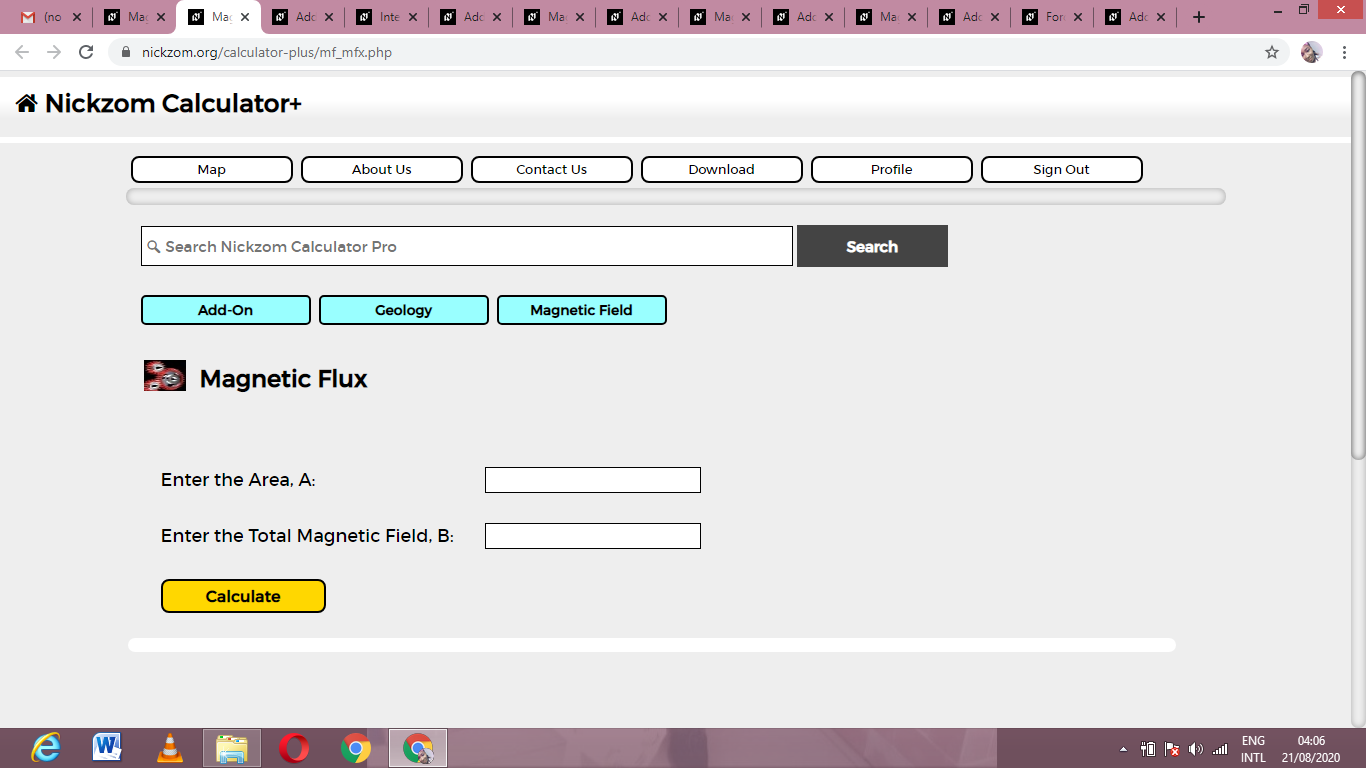Now, enter the values appropriately and accordingly for the parameters as required by the Area (A) is 32 and Total Magnetic Field (B) is 18.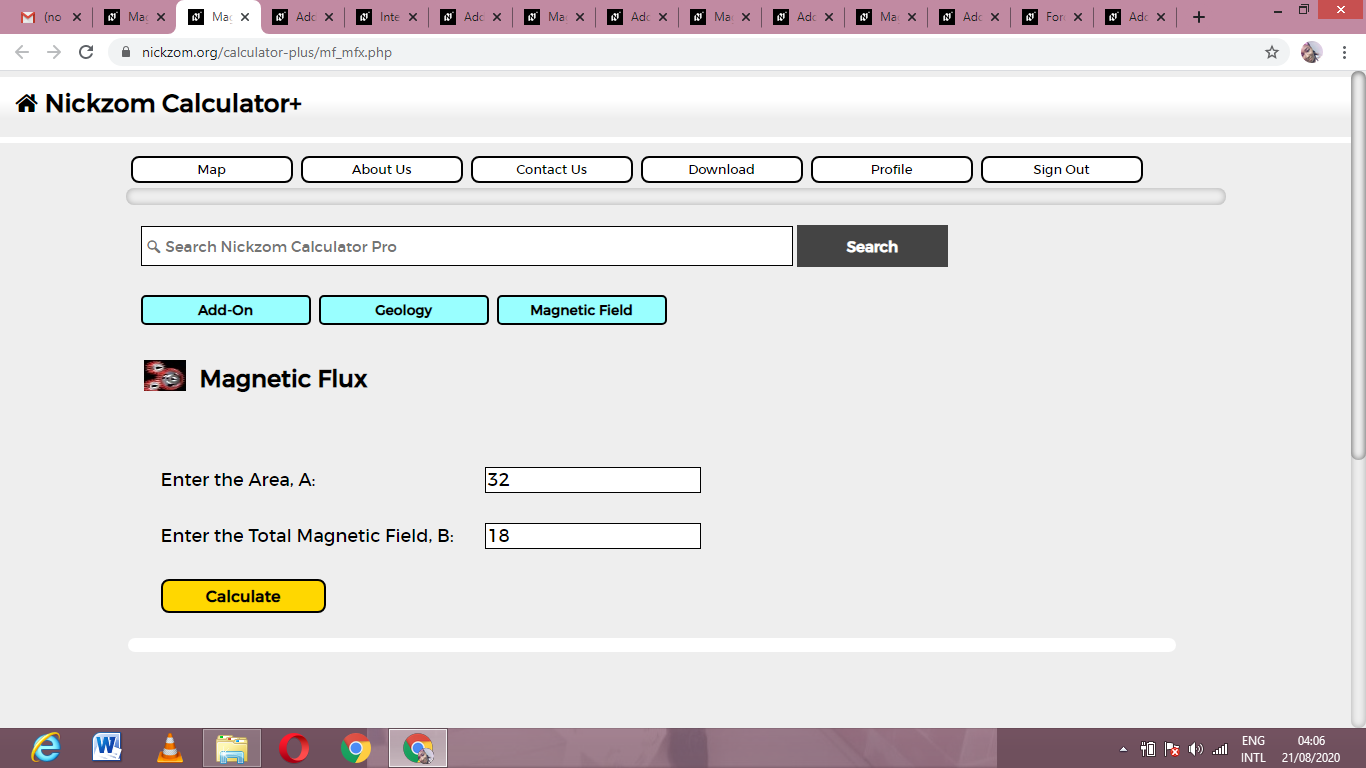Finally, Click on Calculate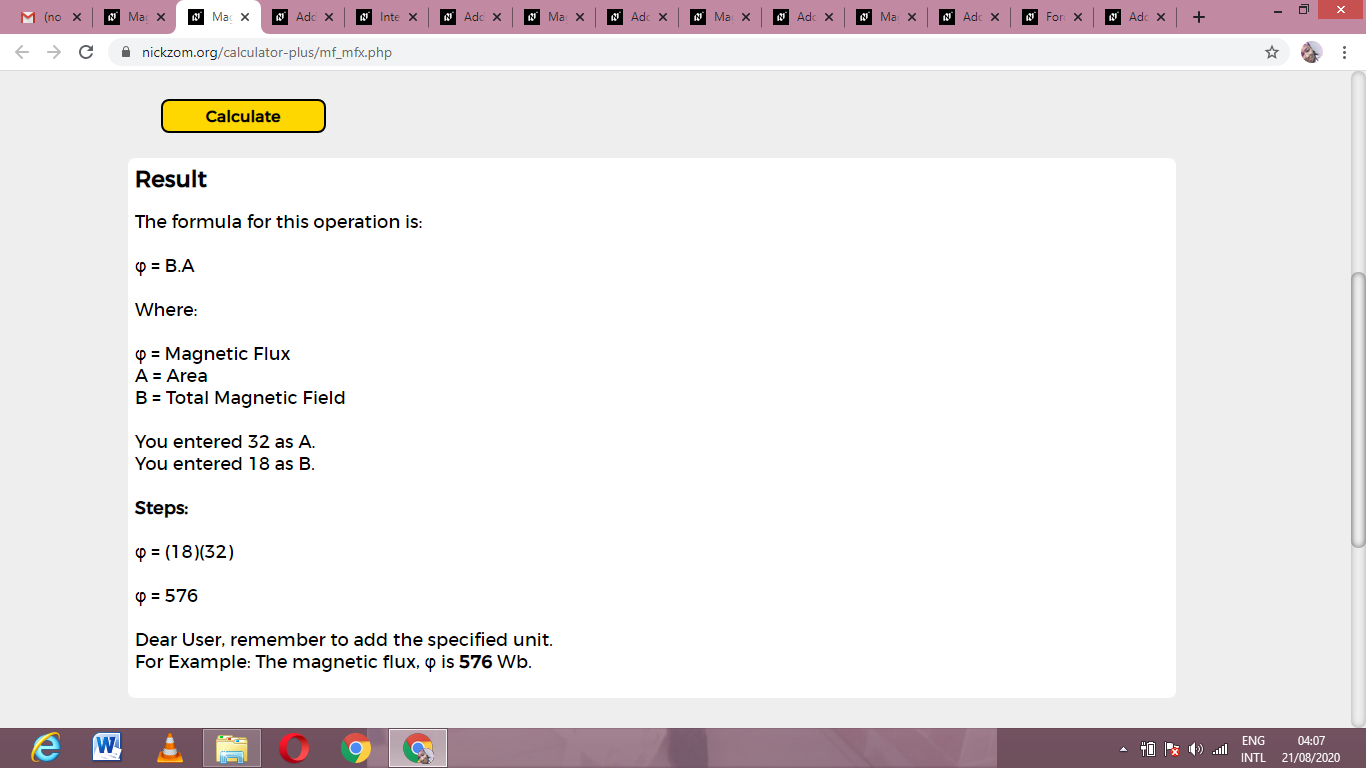As you can see from the screenshot above, Nickzom Calculator– The Calculator Encyclopedia solves for the magnetic flux and presents the formula, workings and steps too.